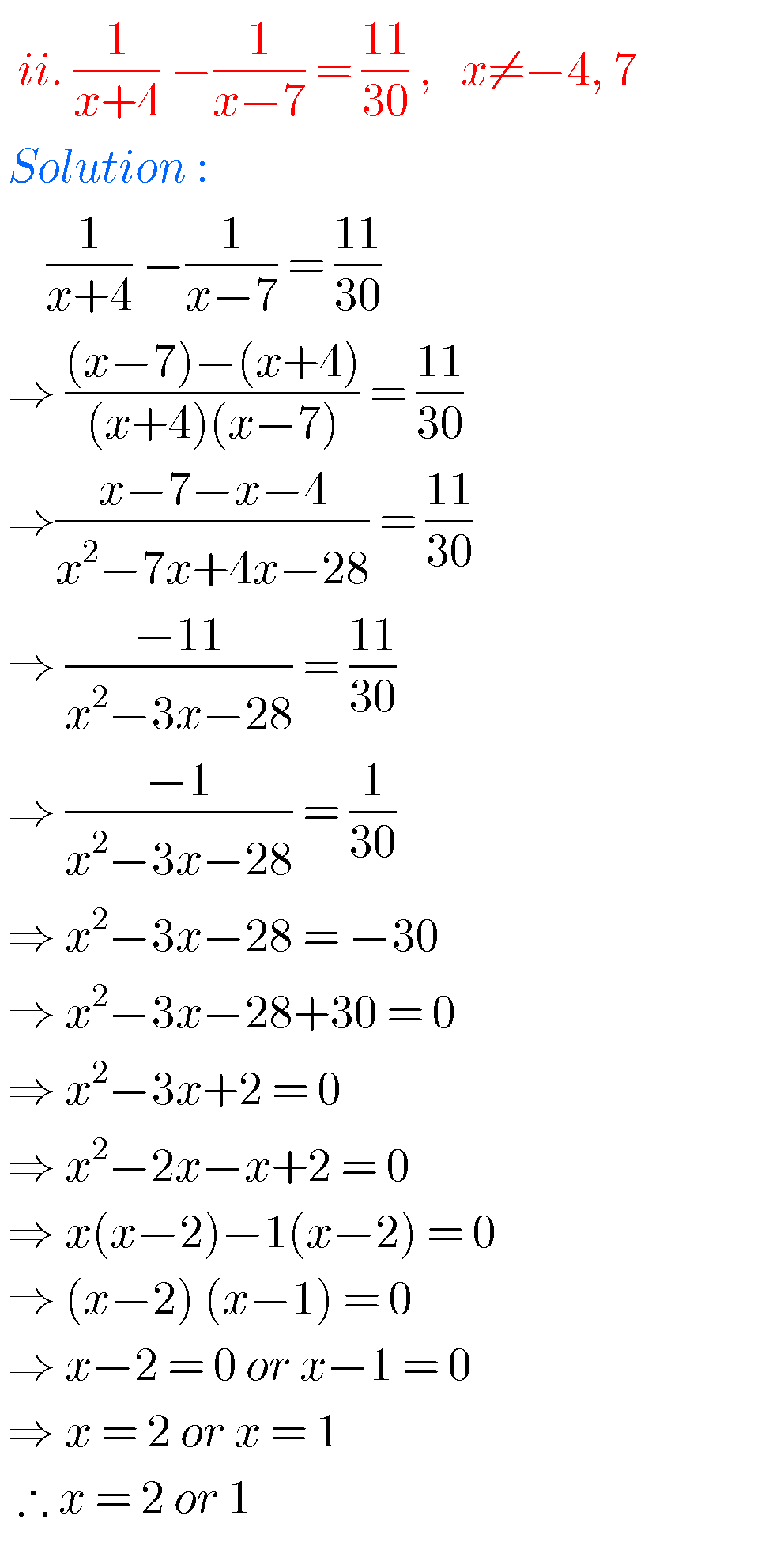## SSC solutions for Maths Quadratic Equations class 10

SSC Class 10 solutions for Maths Quadratic Equations Exercise 5.1,5.2,5.3 and 5.4 Mathematics class 10 SSC solutions for Quadratic equations are given. Exercise 5.1, 5.2. 5.3 and 5.4 problems with solutions are given.  Observe the solutions and try them in your own method. First you study the text book lesson Quadtratic Equations very well.  Observe …